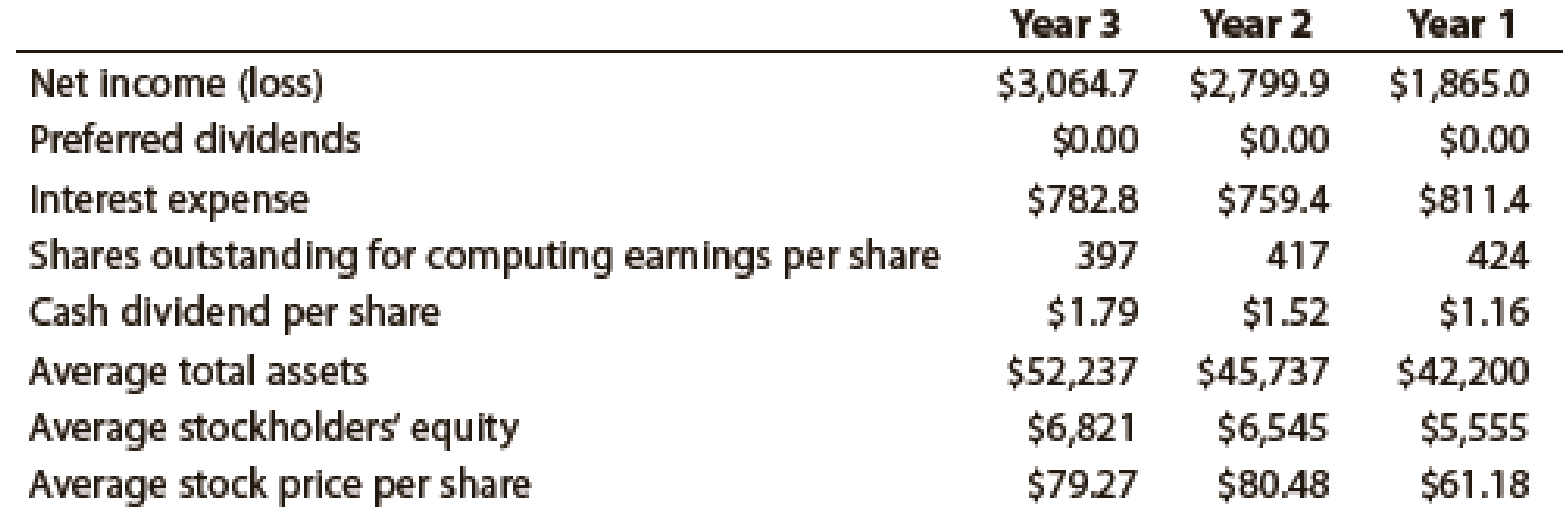Chapter 17, Problem 5CP

Chapter
Section
Textbook Problem
1 views

# Profitability analysisDeere & Company manufactures and distributes farm and construction machinery that it sells around the world. In addition to its manufacturing operations, Deere’s credit division loans money to customers to finance the purchase of their farm and construction equipment.The following information is available for three recent years (in millions except per-share amounts):1. Calculate the following ratios for each year, rounding ratios and percentages to one decimal place, except for per-share amounts: a. Return on total assets b. Return on stockholders’ equity c. Earnings per share d. Dividend yield e. Price-earnings ratio 2. Based on these data, evaluate Deere’s profitability.

To determine

Determine the following ratios for three years:

1. (a) Return on total assets
2. (b) Return on stockholders’ equity
3. (c) Earnings per share
4. (d) Dividend yield
5. (e) Price – earnings ratio
Explanation

a. Return on total assets for three years

Rate of return on assets (Year 3)=Netincome + Interest expenseAverage total assets=$3,064.7+$782.8$52,237=7.4% Rate of return on assets (Year 2)=Netincome + Interest expenseAverage total assets=$2,799.9+$759.4$45,737=7.8%Rate of return on assets (Year 1)=Netincome + Interest expenseAverage total assets=$1,865.0+$811.4$42,200=6.4% Description: Return on assets determines the particular company’s overall earning power. It is determined by dividing sum of net income and interest expense and average total assets. Formula: Rate of return on assets=Netincome + Interest expenseAverage total assets Hence, rate of return on assets for year 3, year 2, and year 1 are 7.4%, 7.8%, and 6.4% respectively. b) Return on stockholders’ equity for three years Rate of return on stockholders' equity(Year 3)}= Net income Average stockholder’s equity=$3,064.7$6,821=44.9% Rate of return on stockholders' equity(Year 2)}= Net income Average stockholder’s equity=$2,799.9$6,545=42.8% Rate of return on stockholders' equity(Year 1)}= Net income Average stockholder’s equity=$1,865.0$5,555=33.6% Description: Rate of return on stockholders’ equity is used to determine the relationship between the net income and the average common equity that are invested in the company. Formula: Rate of return on stockholders' equtiy = Net incomeAverage stockholder’s equity Hence, rate of return on stockholders’ equity for year 3, year 2, and year 1 is 44.9%, 42.8%, and 33.6%. c) Earnings per share on the common stock for three years. Earnings per share (Year 3)=[Net incomePreferred dividends(Weighted average shares of common stock outstanding)]=$3,064.7$0397shares=$7.72Earnings per share (Year 2)=[Net incomePreferred dividends(Weighted average shares of common stock outstanding)]=\$2,799

2.

To determine

Evaluate D’s profitability.

### Still sussing out bartleby?

Check out a sample textbook solution.

See a sample solution

#### The Solution to Your Study Problems

Bartleby provides explanations to thousands of textbook problems written by our experts, many with advanced degrees!

Get Started